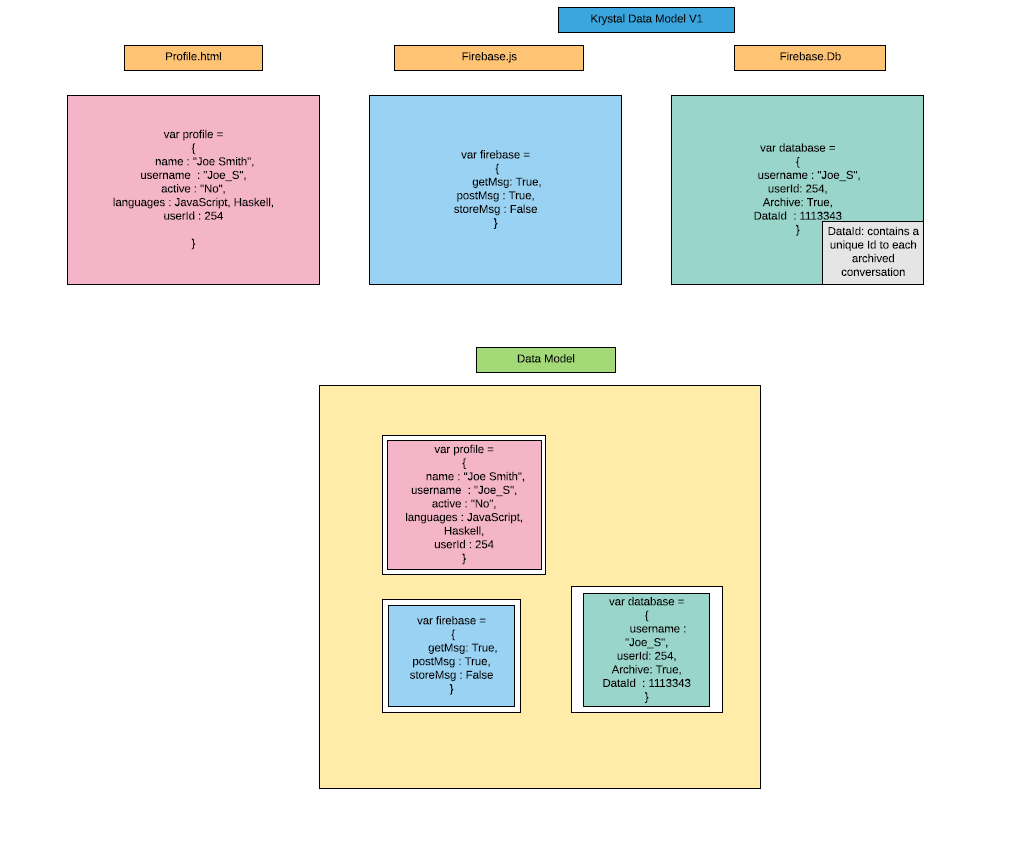# Thursday June 15th

## Data Model, C++ Resources going forward etc• I got some great advice from the people on functional Slack. C++ is such a great language, and it’s challenging, but there is also a lot of stuff that differs from industry, and that’s frustrating.

• I also got a list of good resources :

## Resources :

Gaming Patterns Book

Stack Overflow C++ Book Guide

• Good Youtube C++ tutorial stuff

You Tube C++ Tutorials

Physically Based Rendering pdf

Zach Laine “Pragmatic Type Erasure: Solving OOP Problems with Elegant Design Patterns

## Concepts

RAII : Resource Acquisition is Initialization

## Also

Halide Talk

I had seen this a few months ago, when looking at Recursion for class : DemoScene GPU15

Gameboy talk

## Kata Solved

• Find number couple with greatest difference. Given in form of an array such as :
``````diff(["1-11", "2-3"])
# should return "1-11"

diff(["3-3", "4-4"])

# should return False
either if array is empty
or if the difference is 0
``````

## Solution:

``````def diff(arr):
arr1 = []

# find absolute difference

for i in arr:
arr1.append(abs(eval(i)))

# check that array is not empty, else return False
if len(arr) == 0:
return False

# Find max difference index in original array
else:
b = (max(arr1))

if b != 0:
c = arr1.index(b)
return arr[c]
else:
return False
``````

## Another

• If number of lowercase > uppercase, switch entire string to lowercase, else uppercase entire string

## Solution

``````def case_unification(n):
l = 0
u = 0

for i in n:
if i == i.lower():
l = l + 1
elif i == i.upper():
u = u + 1

if l > u:
return n.lower()
else:
return n.upper()
``````

## Another

• Find even numbers before a value in an array

• If value not in array, return -1. If no value, return 0, etc.

## Solution

``````function evenNumbersBeforeFixed(sequence, fixedElement) {
var total = 0;
var arr = []
if (!(sequence.includes(fixedElement) === true)){
return(-1)
}

var a = sequence.indexOf(fixedElement)
//console.log(a)
for (var i = 0; i < a; i++){
if (sequence[i] % 2 === 0)
{
total = total + 1;
//arr.push(total)
}
}

}
``````

## Kata

Fit format to match (n) -> “R\$ 0,00”

## Solution

``````function currencyBRL(number) {
if (number == null){
var a = 'R\$ 0,00'
}
else{
var a = ('R\$ ' + number.toFixed(2).replace(/(\d)(?=(\d{3})+\.)/g, '\$1,'));
var a = a.replace(".", ",");
}
return a
}
``````

Should have done that in one regex but…life goals :)

## Something about Haggling in Bali…Solution :

``````function vestBuy(price, haggle)
{
if (haggle == "light"){
return(.80 * price)
}
else if (haggle == "medium"){
return(.70 * price)
}
else if (haggle == "heavy"){
return(.60 * price)
}
else if (haggle == "walkandswear"){
return(.10 * price)
}
else{
return("Run!!")
}
return
}
``````

## AI (Articial Intelligence/ Data Science)

• I had started an Artificial Intelligence / Data Science course. We’ll see how it goes. It’s free for now, so why not? I may just become a data-scientist! :)